The VARMAX Procedure

Bayesian VAR and VARX Modeling

Subsections:

Consider the VAR(p) model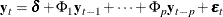or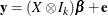When the parameter vectorhas a prior multivariate normal distribution with known mean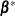and covariance matrix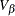, the prior density is written as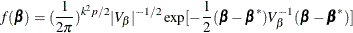The likelihood function for the Gaussian process becomes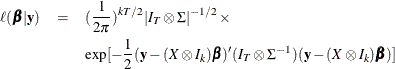Therefore, the posterior density is derived as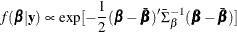where the posterior mean is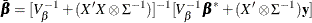and the posterior covariance matrix is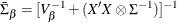In practice, the prior meanand the prior varianceneed to be specified. If all the parameters are considered to shrink toward zero, the null prior mean should be specified. According to Litterman (1986), the prior variance can be given by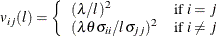where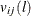is the prior variance of the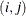th element of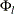,is the prior standard deviation of the diagonal elements of,is a constant in the interval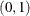, and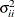is the ith diagonal element of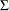. The deterministic terms have diffused prior variance. In practice, you replace theby the diagonal element of the ML estimator ofin the nonconstrained model.

For example, for a bivariate BVAR(2) model,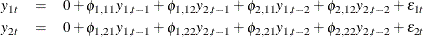with the prior covariance matrix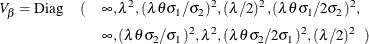For the Bayesian estimation of integrated systems, the prior mean is set to the first lag of each variable equal to one in its own equation and all other coefficients at zero. For example, for a bivariate BVAR(2) model,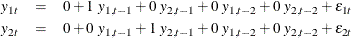Forecasting of BVAR Modeling

The mean squared error (MSE) is used to measure forecast accuracy (Litterman, 1986). The MSE of the s-step-ahead forecast is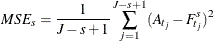where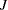is the number specified by NREP= option,is the time index of the observation to be forecasted in repetition j,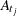is the actual value at time, and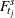is the forecast made s periods earlier.

Bayesian VARX Modeling

The Bayesian vector autoregressive model with exogenous variables is called the BVARX(p,s) model. The form of the BVARX(p,s) model can be written as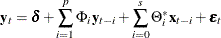The parameter estimates can be obtained by representing the general form of the multivariate linear model,The prior means for the AR coefficients are the same as those specified in BVAR(p). The prior means for the exogenous coefficients are set to zero.

Some examples of the Bayesian VARX model are as follows:

   model y1 y2 = x1 / p=1 xlag=1 prior;

   model y1 y2 = x1 / p=(1 3) xlag=1 nocurrentx
prior=(lambda=0.9 theta=0.1);